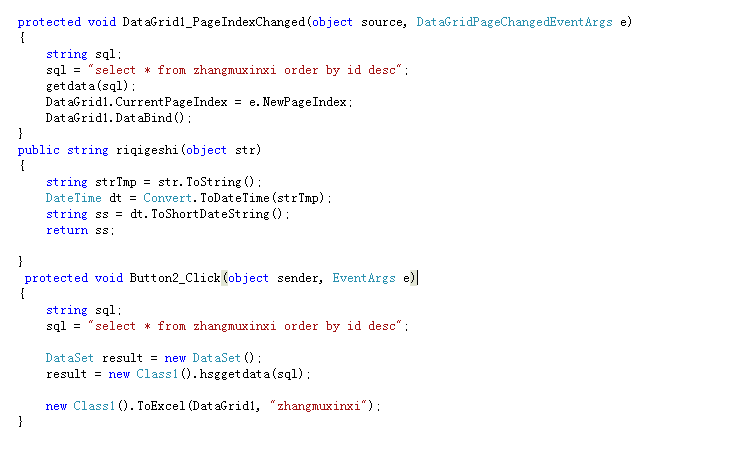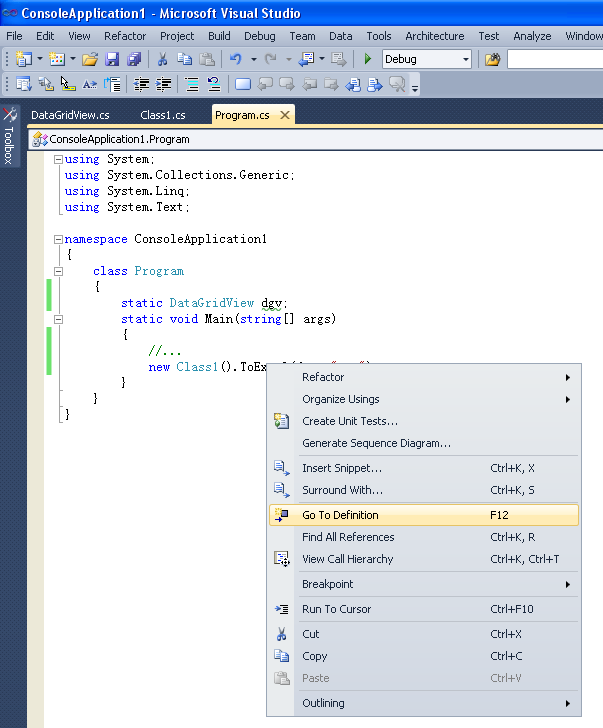C#项目，将报表导出成Excel文件，这个是怎么实现的？3个回答

vs环境，代码里光标选择Class1，点右键，点转到定义Windows 7，Visual Studio 2010， Microsoft Office 2007

C#创建Excel文件，这里实际上是从资源中提取一个事先创建好的Excel文件，文件提取成功后，使用OleDb方法连接Excel，向Excel文件中写入数据。

[csharp] view plain copy

string excelPath = AppDomain.CurrentDomain.BaseDirectory + "Excel" + DateTime.Now.Ticks + ".xlsx";

if (System.IO.File.Exists(excelPath))

{

textBox1.Text += ("文件已经存在！");

return;

}

try

{

//从资源中提取Excel文件

System.IO.FileStream fs = new System.IO.FileStream(excelPath, FileMode.OpenOrCreate);

fs.SetLength(0);

fs.Write(Properties.Resources.Excel, 0, Properties.Resources.Excel.Length);

fs.Close();

fs.Dispose();

textBox1.Text = "提取Excel文件成功！" + "\r\n";

}

catch (System.Exception ex)

{

excelPath = string.Empty;

textBox1.Text += ("提取Excel文件失败：" + ex.Message);

textBox1.Text += ("\r\n");

Application.DoEvents();

return;

}

[csharp] view plain copy

//定义OleDB连接字符串

string strConn = "Provider=Microsoft.Ace.OleDb.12.0;Persist Security Info=False;" + "data source=" + @excelPath + ";Extended Properties='Excel 12.0; HDR=yes; IMEX=10'";

OleDbConnection conn = new OleDbConnection();

conn.ConnectionString = strConn;

[csharp] view plain copy

DataTable oleDt = conn.GetOleDbSchemaTable(OleDbSchemaGuid.Tables, null);

dataGridView1.DataSource = oleDt;

dataGridView1.Show();

[csharp] view plain copy

OleDbCommand cmd = null;

try

{

//向"Sheet1"表中插入几条数据,访问Excel的表的时候需要在表名后添加"\$"符号,Insert语句可以不指定列名

cmd = new OleDbCommand("Insert Into [Sheet1\$] Values('abc', 'bac', '0', '123456', 'test','测试','aa')", conn);//(A,B,C,D,E,F,G)

cmd.ExecuteNonQuery();

cmd.ExecuteNonQuery();

cmd.ExecuteNonQuery();

cmd.ExecuteNonQuery();

cmd.ExecuteNonQuery();

}

catch (System.Exception ex)

{

textBox1.Text += ("插入数据失败：" + ex.Message);

textBox1.Text += ("\r\n");

}

[csharp] view plain copy

cmd = new OleDbCommand("Select * From [Sheet1\$]", conn);

DataSet ds = new DataSet();

dataGridView2.DataSource = ds.Tables;

[csharp] view plain copy

DataTable dt = conn.GetSchema();

for (int i = 0; i < dt.Columns.Count; i++)

{

textBox1.Text += dt.Columns[i].Caption;

if (i + 1 < dt.Columns.Count)

{

textBox1.Text += ",";

}

}

for (int j = 0; j < dt.Rows.Count; j++)

{

for (int i = 0; i < dt.Columns.Count; i++)

{

if (dt.Rows[j][dt.Columns[i]] != null)

{

textBox1.Text += dt.Rows[j][dt.Columns[i]].ToString();

}

else

{

textBox1.Text += "null";

}

``````    if (i + 1 < dt.Columns.Count)
{
textBox1.Text += ",";
}
}
textBox1.Text += ("\r\n");
``````

}

[csharp] view plain copy

if (conn.State != ConnectionState.Closed)

{

try

{

conn.Close();

}

catch (System.Exception ex)

{

textBox1.Text += ("关闭Excel数据连接：" + ex.Message);

textBox1.Text += ("\r\n");

}

}

[csharp] view plain copy

System.Diagnostics.Process.Start("explorer.exe", AppDomain.CurrentDomain.BaseDirectory);